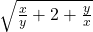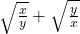# TS Polycet (Polytechnic) 2016 Previous Question Paper with Answers And Model Papers With Complete AnalysisTS Polycet (Polytechnic) 2016 Previous Question Paper with Answers And Model Papers With Complete Analysis
TS Polycet (Polytechnic) Previous Year Question Papers And Model Papers:
While preparing for TS Polycet (Polytechnic), candidates must also refer to the previous year question papers of the same. Scoring well in TS Polycet (Polytechnic) and understanding weaknesses and strengths in the respective sections.

TS Polycet (Polytechnic) Previous Year Question Papers can be found on this page in PDF format. Students taking the exam to get into some of the best Polytechnic colleges/institutes in the state of Andhra Pradesh may practice these papers to get a clear idea of the structure of the exam, marking scheme, important topics, etc.

## Section — I MATHEMATICS

Q). The relation a . (b . c) = (a . b) . c is
A) distributive law
B) associative law
C) commutative law
D) closure law

B) associative law

Q). The HCF of 1.2 .and 0.12 is
A) 1.2
B) 12
C) 0.12
D) 120

C) 0.12

Q). The value of log3 243 =
A) 5
B) 6
C) 4
D) 8

A) 5

Q). 0.10 110 1110 11110 ……………is
A) an integer
B) a rational number
C) an irrational number
D) a natural number

C) an irrational number

Q). If x = log2 3 and y = log2 5, then log2 15 in terms of x and y is
A) x – y
B) x + y
C) xy
D) x + y – 1

B) x + y

Q). If A ⊂ B and B ⊂ C, then (A ∩ B) ∪ C =
A) Ø
B) A
C) B
D) C

D) C

Q). If A = {x l x ∈ N, 3 ≤ x ≤ 6], then A =
A) {3, 4, 5}
B) {3, 4, 5, 6}
C) {4, 5}
D) { 4, 5, 6}

B) {3, 4, 5, 6}

Q). If A = (x I x is an even natural number less than 12} and B = (x I x is a prime numberless than 12}, then A ∩ B =
A) {2, 4. 6, 8,10}
B) {2, 3, 5, 7}
C) Ø
D) {2}

D) {2}

Q). If two zeroes of the polynomial x3 -5x2 + 6x are 2 and 3, then the third is
A) 0
B) 5
C) 6
D) 1

Q). The value of=
C)C)